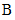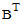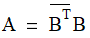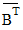# NMath User's Guide

20.1 Factorization Classes (.NET, C#, CSharp, VB, Visual Basic, F#)

The factorization classes associated with each matrix type are shown in Table 17.

 Matrix Classes Factorization Classes SymmetricMatrix SymFact SymPDFact HermitianMatrix HermitianFact HermitianPDFact BandMatrix BandFact TriDiagMatrix TriDiagFact SymPDTriDiagFact HermPDTriDiagFact SymBandMatrix SymPDBandFact HermitianBandMatrix HermitianPDBandFact

Note that lower and upper triangular types do not have factorization classes; these types are typically the result of factoring other matrices (for example, into the product of a lower and upper triangular matrix). Static methods for solving for different right-hand sides, and computing inverses, determinants, and condition numbers, are provided on class MatrixFunctions for triangular types.

Note also that NMath provides two factorization classes for symmetric and Hermitian types: one for indefinite matrices, and one for positive definite (PD) matrices. A symmetric matrixis positive definite if there exists a nonsingular matrixsuch that:whereis the transpose of. A Hermitian matrix is positive definitive if there exists a nonsingular matrixsuch that:whereis the conjugate transpose of. Positive definite matrices arise frequently in statistical applications.

If you don't know whether a particular symmetric or Hermitian matrix is positive definite, the easiest way to find out in NMath is to attempt to factor the matrix using the associated PD factorization class (Section 20.2). The read-only property IsPositiveDefinite returns true if the given matrix is positive definite and the factorization can be used to solve equations, compute determinants, inverses, and so on.

Top

Top